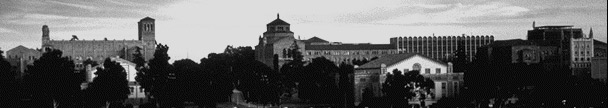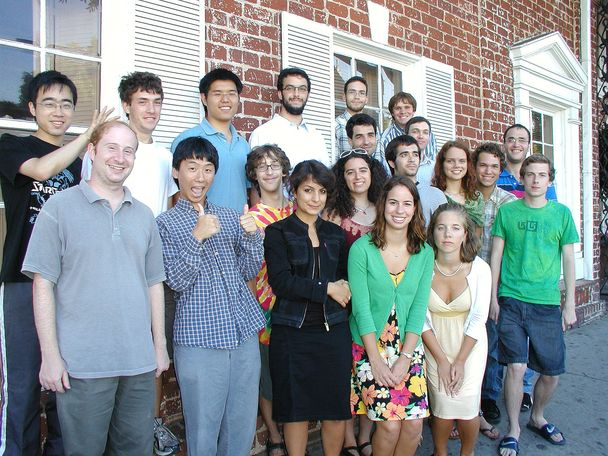UCLA Logic Center# 2009 Summer School for Undergraduates

## (This is the 2009 page. Are you looking for the current Summer School?)

The UCLA Logic Center held a three-week summer school for undergraduates, from Monday July 13, to Friday July 31, 2009. (Summer school flyer, 8.5 x 11 inches, PDF format.)

The goal of the center's summer schools is to introduce future mathematicians to central results and research techniques from mathematical logic. Courses are very intensive, designed to assume little if any prior experience with logic, yet reach advanced graduate material within three weeks. They serve as good introduction to the kind of research work that students of mathematics can expect in graduate school.

Thanks to an NSF grant and Logic Center support, we were able to provide admitted students with a stipend of \$3000, a travel allowance up to \$500, and dormitory housing at UCLA for no charge.

Courses in the summer school meet daily for two hours of lecture, and one hour of guided problem solving. Students are asked to select two of the three courses offered. In addition to the six daily hours of course work, there are lectures on topics of current research, social events, and planned outings in the area.### Topics in 2009:

First-order logic and Gödel's incompleteness theorem
Instructor: Henry Towsner

At the end of the nineteenth century, mathematicians believed they were closing in on a system of formal mathematical rules powerful enough to answer any question of mathematics. Instead, they came upon the Incompleteness Theorem: Gödel's proof that attempts to formalize mathematics must leave some questions unanswered. In other words, mathematics, and the philosophy of mathematics, can never be finished, since any attempt to give the "rules" of mathematics will lead to questions which require new rules to answer. In order to prove the theorem, we will give precise meaning to ideas like "mathematical rules" and "proofs": the framework of first-order logic. Along the way, we'll discover other properties of first-order logic, which will tell us more about both the expanse and the limits of mathematical reasoning.

Forcing and independence in set theory
Instructor: Justin Palumbo

Set-theoretic forcing is a technique originally introduced by Paul Cohen to prove that the axiom of choice and the continuum hypothesis are independent of the classical axioms of mathematics. Since then it has led to an explosive growth in research, and has been used by set theorists to prove literally hundreds of independence results. In this course we will introduce students to the forcing technique by first studying some of the combinatorial consequences of Martin's Axiom, an axiom which when added to ZFC makes it possible to prove consistency results by means very similar to those used in forcing. We will then introduce forcing itself and show how it can be applied to settle the independence of the continuum hypothesis.

Non-standard analysis
Instructor: Isaac Goldbring

Infinitely small numbers and infinitely large numbers have been used as a heuristic tool in mathematics throughout the centuries: Archimedes used them to derive formulas for the areas and volumes of geometric figures, Newton and Leibniz used infinitesimals to develop the differential and integral calculus, and Euler employed infinitesimals to derive series expansions of logarithmic, exponential, and trigonometric functions. But the concept remained vague, and its use became unacceptable with the introduction of a rigorous foundation for analysis in the 19th century. In the early 1960s, the logician Abraham Robinson realized that techniques from mathematical logic, most notably the compactness theorem, could be used to give infinitesimal analysis a firm foundation. In this course, we will develop Robinson's rigorous non-standard methods, and use them to prove theorems from a wide spectrum of mathematical areas, including basic analysis, metric space topology, measure theory, functional analysis, and combinatorics.